[单例模式]- ESM / CommonJS 规范

Java 中的单例模式

public class SingleObject {

//创建 SingleObject 的一个对象
private static SingleObject instance = new SingleObject();

//让构造函数为 private，这样该类就不会被实例化
private SingleObject(){}

//获取唯一可用的对象
public static SingleObject getInstance(){
return instance;
}

public void showMessage(){
System.out.println("Hello World!");
}
}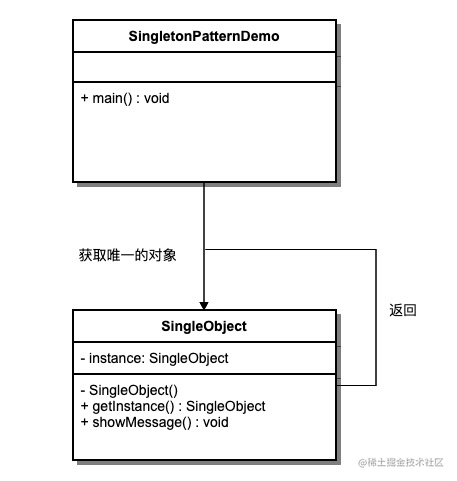JavaScript 的实现

基于 Java 思想的单例

const SingletonPerson = function(name, age) {
this.name = name;
this.age = age;
}

SingletonPerson.getInstance = function(name, age) {
if(!this.instance) {
this.instance = new SingletonPerson(name, age);
}
return this.instance;
}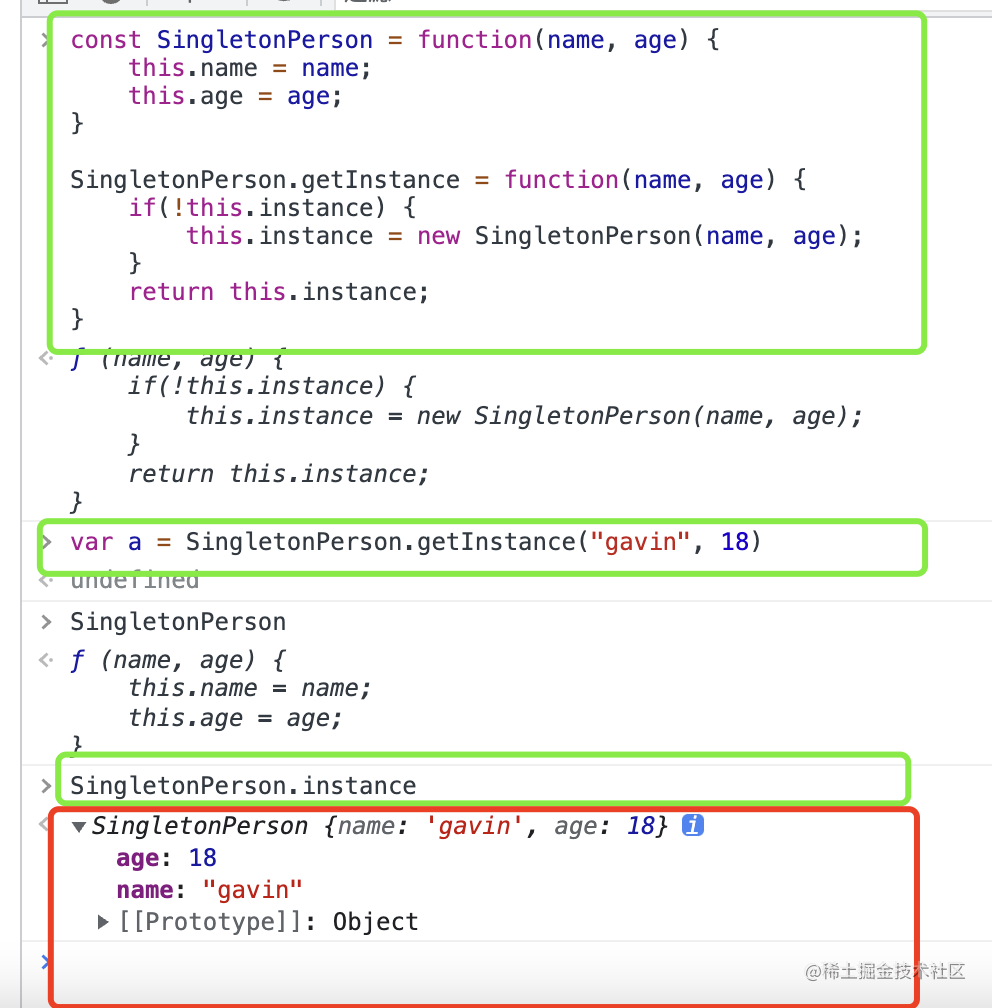\

• 这个实例其实是挂载在 SingletonPerson 这个函数上的 instance 上的，对于我们的理解，可能会有一定的问题；
• 我们的创建方法，需要显示地调用 getInstance 这个函数。

\

一个普通的 JS 单例

const getInstance = (function(){
let instance = {
name: "luyi"
};
return function() {
return instance;
}
})();

const a = getInstance();
const b = getInstance();

console.log( a === b );

一个透明的单例

const SingletonPerson = (function() {
const Person = function(name, age) {
this.name = name;
this.age = age;
}
let instance = null;
return function(name, age) {
if(!instance) {
instance = new Person(name, age);
}
return instance;
}

})();

const a = new SingletonPerson("gavin",18);
const b = new SingletonPerson("luyi",19);
console.log(b, a===b);  //Person {name: 'gavin', age: 18} , true

一个单例化的函数

const Person = function (name, age) {
this.name = name;
this.age = age;
}

const singlelify = function (Func) {
let instance = null;
return function (...args) {
if (!instance) {
instance = new Func(...args);
}
return instance;
}
};

const SigletonPerson = singlelify(Person);
const a = SigletonPerson("gavin", 20);
const b = SigletonPerson("luyi", 19);

console.log(b, a === b)  //Person {name: 'gavin', age: 20}, true

模块化中的单例与闭包思想

cjs 中的单例体现

// single.js
let count = 0;

function getCount() {
return count++;
}

module.exports = {getCount};
// index.js
const a = require('./single').getCount();
const b = require('./single').getCount();
const c = require('./single').getCount();

console.log(a, b, c)
// 这里打印的结果是，0，1，2

./node_modules/.bin/webpack --entry ./index.js -o output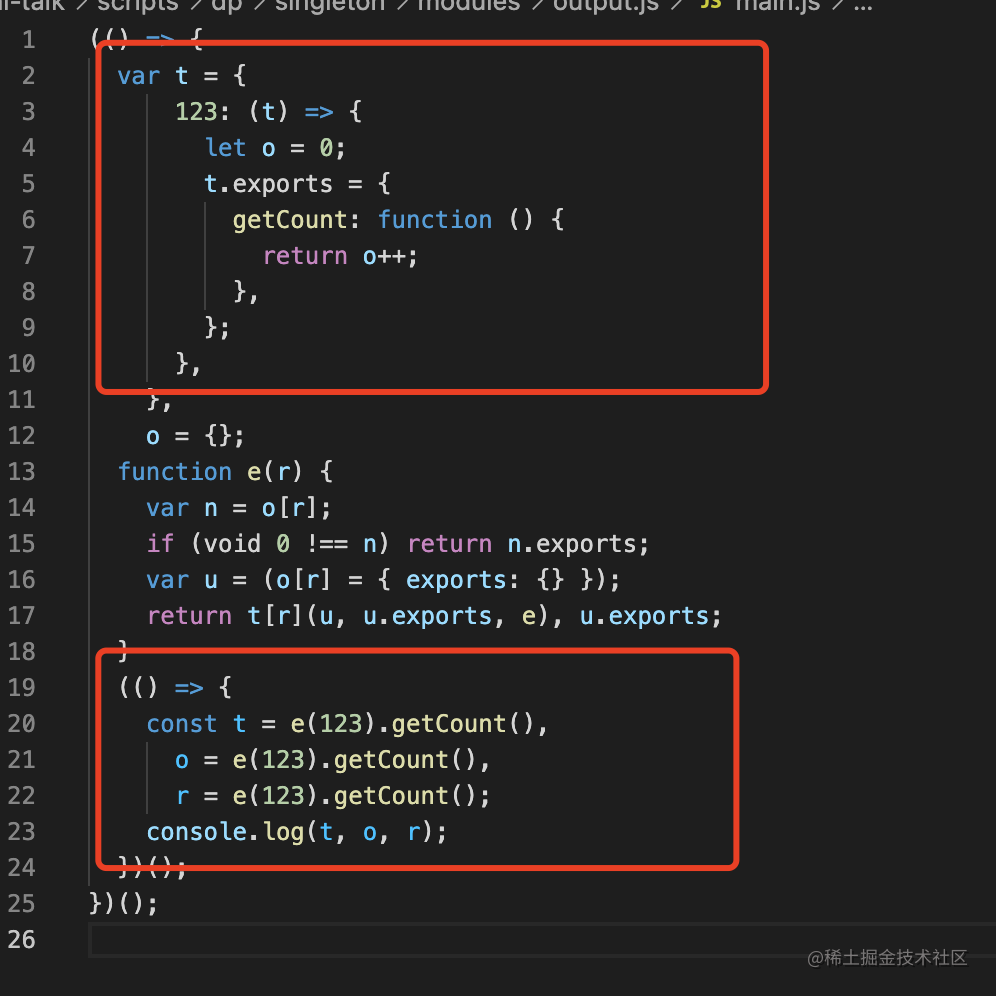// single.js
let instance = null;
function Person(name, age) {
this.name= name;
this.age = age;
}

function getInstance(name, age) {
if(!instance) {
instance = new Person(name, age);
}
return instance
}

module.exports = {getInstance};

// index.js

const a = require('./single').getInstance("luyi", 18);
const b = require('./single').getInstance("gavin", 19);
const c = require('./single').getInstance("candy", 20);

console.log(a, b, c, a===c)
// 这里打印的结果是，
// Person { name: 'luyi', age: 18 }
// Person { name: 'luyi', age: 18 }
// Person { name: 'luyi', age: 18 }
// true

esm 中的单例体现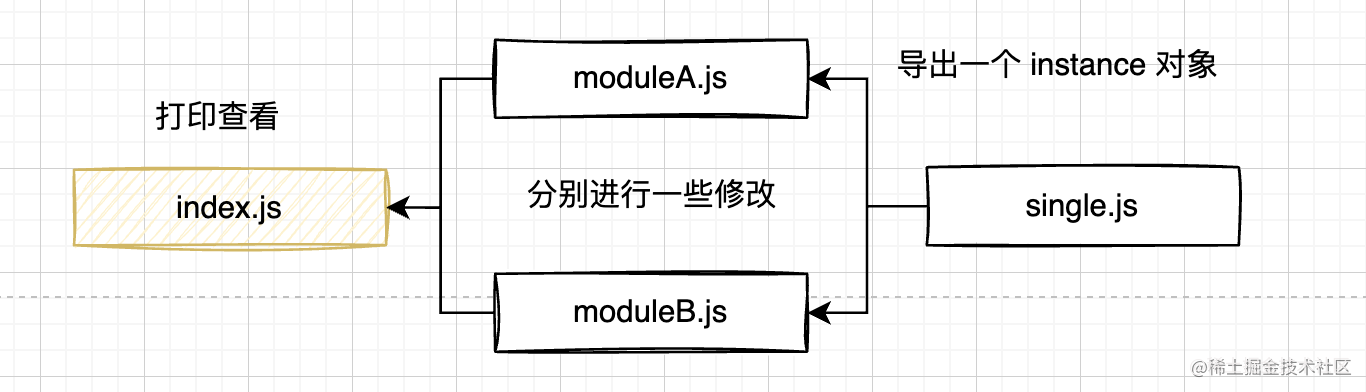// single.js
function Person(name, age) {
this.name= name;
this.age = age;
}
let _instance = new Person("luyi", 19);
export const instance = _instance;

// moduleA.js
import { instance } from "./single";
instance.age = 30;
export const instanceA = instance;

// moduleB.js
import { instance } from "./single";
instance.name = "gavin"
export const instanceB = instance;

// index.js
import { instanceA } from "./moduleA";
import { instanceB } from "./moduleB";
console.log(instanceA,instanceB,instanceA === instanceB);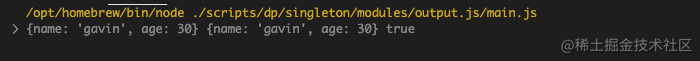总结

参考资料

JavaScript设计模式与开发实践